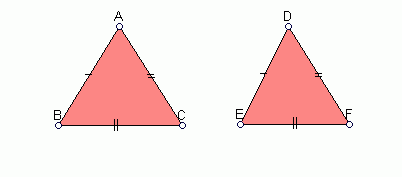Email us to get an instant 20% discount on highly effective K-12 Math & English kwizNET Programs!

#### Online Quiz (WorksheetABCD)

Questions Per Quiz = 2 4 6 8 10

### Geometry2.20 Congruency of Triangles

 Congruent Figures Two plane figures are said to congruent if they have the same shape and same size. Two line segments having the same length are congruent.Congruent Triangles There are four properties of congruency in triangles. The SAS Property: If two sides and the included angle of a triangle are respectively equal to two sides and the included angle of another triangle, then the two triangles are congruent. Let us consider two trianglesABC andDEF.Conditions: If AB = DE, BC = EF and the included angle BAC = the included angle EDF. If the above conditions are satisfied, then we say that the two trianglesABC andDEF are congruent and we can write it asABC @DEF. This is called Side, Angle, Side property. The ASA Property: If two angles and a side of one triangle are respectively equal to two angles and the corresponding side of another triangle, then the two triangles are congruent. Let us consider two triangles ABC and DEF.Conditions: If BC = EF, �B = �E and �C = �F. If the above conditions are satisfies then we say that the two triangles ABC and DEF are congruent and we can write it asABC @DEF. This is called Angle, Side, Angle property. The SSS Property: If three sides of a triangle are equal to the three sides of another triangle, then the two triangles are congruent. Let us consider two triangles ABC and DEF.Conditions: If AB = DE, BC = EF AND AC = DF. If the above conditions are satisfies then we say that the two triangles ABC and DEF are congruent and we can write it asABC @DEF. This is called Side, Side, Side property. The RHS Property: If the hypotenuse and a side of a right-angled triangle are equal to the hypotenuse and a side of another right-angled triangle, then the two triangles are congruent. Let us consider two triangles ABC and DEF.Conditions: �B = �E = 90�, side BC = Side EF and hypotenuse AC = hypotenuse DF. If the above conditions are satisfies then we say that the two triangles ABC and DEF are congruent and we can write it asABC @DEF. This is called the Right Angle, Hypotenuse, Side property. Directions: Read the above review points carefully and answer the following questions: Illustrate each of the above properties by giving at least 5 examples. Explain in your own words the different properties of congruent triangles. Explain why there is no ASS property for congruent triangles.
 Q 1: Two circles are congruent if they have the same ______.centerradiusarc Q 2: Two line segments are congruent if they have the same _____.arealengthshape Q 3: Two line segments having the same length are ______.equalcongruent Q 4: Two plane figures are said to be congruent if they have same size and same _____.lengthshapeangle Q 5: If two sides and the included angle of a triangle are respectively equal to two sides and the included angle of another triangle, then the two triangles are congruent.SAS PropertySSS PropertyRHS PropertyASA Property Q 6: If three sides of a triangle are equal to the three sides of another triangle, then the two triangles are congruent.RHS PropertySAS PropertySSS PropertyASA Property Question 7: This question is available to subscribers only! Question 8: This question is available to subscribers only!